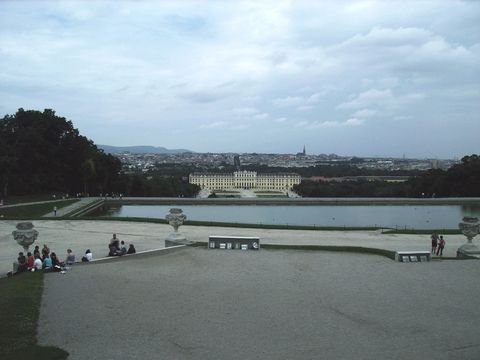## Tweak

`Tweak `(clip, float "hue", float "sat", float "bright", float "cont", bool "coring", bool "sse", float "startHue", float "endHue", float "maxSat", float "minSat", float "interp")

This function provides the means to adjust the hue, saturation, brightness, and contrast of a video clip. In v2.58, both the saturation and hue can be adjusted for saturations in the range [minSat, maxSat] and hues in the range [startHue, endHue]. interp interpolates the adjusted saturation to prevent banding.

Hue: (-180.0 to +180.0, default 0.0) is used to adjust the color hue of the image. Positive values shift the image towards red. Negative values shift it towards green.

Sat: (0.0 to 10.0, default 1.0) is used to adjust the color saturation of the image. Values above 1.0 increase the saturation. Values below 1.0 reduce the saturation. Use sat=0 to convert to grayscale.

Bright: (-255.0 to 255.0, default 0.0) is used to change the brightness of the image. Positive values increase the brightness. Negative values decrease the brightness.

Cont: (0.0 to 10.0, default 1.0) is used to change the contrast of the image. Values above 1.0 increase the contrast. Values below 1.0 decrease the contrast.

coring = true/false (optional; true by default, which reflects the behaviour in older versions) is added. When setting to true is means that the luma (Y) is clipped to [16,235], and when setting to false it means that the luma is left untouched. [Added in v2.53.]

sse = true/false (optional; false by default) re-enables the SSE code if required (perhaps an AMD might run it faster). [Added in v2.56.]

startHue (default 0), endHue (default 360): (both from 0 to 360; given in degrees.). The hue and saturation will be adjusted for values in the range [startHue, endHue] when startHue<endHue. Note that the hue is periodic, thus a hue of 360 degrees corresponds with a hue of zero degrees. If endHue<startHue then the range [endHue, 360] and [0, startHue] will be selected (thus anti-clockwise). If you need to select a range of [350, 370] for example, you need to specify startHue=370 and endHue=350. Thus when using the default values all pixels will be processed.

maxSat (default 150), minSat (default 0): (both from 0 to 150 with minSat<maxSat; given in percentages). The hue and saturation will be adjusted for values in the range [minSat, maxSat]. Practically the saturation of a pixel will be in the range [0,100] (thus 0-100%), since these correspond to valid RGB pixels (100% corresponds to R=255, G=B=0, which has a saturation of 119). An overshoot (up to 150%) is allowed for non-valid RGB pixels (150% corresponds to U=V=255, which has a saturation of sqrt(127^2+127^2) = 180). Thus when using the default values all pixels will be processed.

interp: (0 to 32, default 16) is used to interpolate the adjusted saturation. The interpolation is done in the range [minSat-interp, minSat] and [maxSat, maxSat+interp]. There is no interpolation for interp=0, which can be useful when a clip consists of uniform colors. The interpolation is linear.

### Usage and examples: adjusting contrast and brightnessoriginal

There are two problems with this picture. It is too dark, and the contrast is too small (the details of the tree are not visible for example). First, we will increase the brightness to make the picture less dark (left picture below). Second, we will increase the contrast to make details in the dark areas more visible (right picture below). Make sure that the bright parts don't get too bright though.bright=20 bright=20, cont=1.2

### Usage and examples: adjusting saturationoriginal sat=0.75

Suppose we want to lower the saturation of the skin of the girl, and the background should be left intact. The proper way to do this is to set sat=0, and lower the hue range till you found the correct hue range which should be processed. This can be done by using a Vectorscope, but also manually. (If it is needed the saturation range can also be specified to if the dark and white parts of that hue should not be processed.) The result is below.sat=0, startHue=105, endHue=138 sat=0.75, startHue=105, endHue=138

Instead, we can also try to "select" the skin of the girl by narrowing the saturation range only. The result is below. In this case the result is pretty much identical.sat=0, maxSat=75, minSat=55 sat=0.75, maxSat=75, minSat=55

Changelog:

 v2.56 added sse=true/false to enable sse code v2.58 added startHue, endHue, maxSat, minSat and interp

\$Date: 2007/08/22 20:51:54 \$